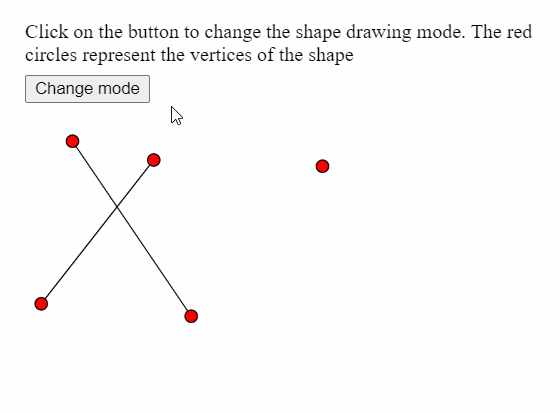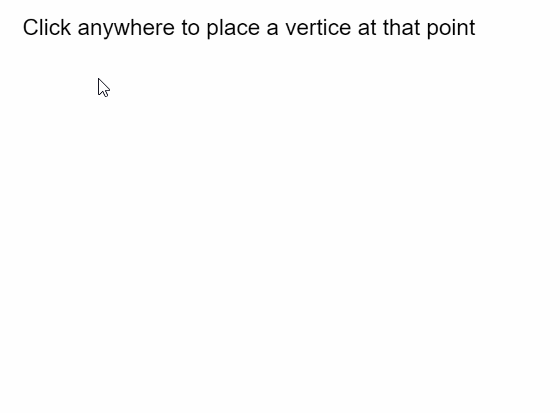# p5.js | vertex() Function

• Last Updated : 31 May, 2020

The vertex() function in p5.js is used to specify the coordinates of the vertices used to draw a shape. It can only be used with the beginShape() and endShape() functions to make various shapes and curves like points, lines, triangles, quads and polygons.

Syntax:

`vertex( x, y )`

OR

`vertex( x, y, z, [u], [v] )`

Parameters: This function accepts five parameters as mentioned above and described below:

• x: It is a number that specifies the x-coordinate of the vertex.
• y: It is a number that specifies the y-coordinate of the vertex.
• z: It is a number that specifies the z-coordinate of the vertex.
• u: It is a number that specifies the u-coordinate of the texture of the vertex. It is an optional parameter.
• v: It is a number that specifies the v-coordinate of the texture of the vertex. It is an optional parameter.

The examples below illustrates the vertex() function in p5.js:

Example 1:

 `let currMode;`` ` `function` `setup() {``  ``createCanvas(400, 300);``  ``textSize(18);`` ` `  ``let shapeModes = [LINES, TRIANGLES, TRIANGLE_FAN, ``                    ``TRIANGLE_STRIP, QUADS];``  ``let index = 0;``  ``currMode = shapeModes[index];`` ` `  ``let helpText = createP(``    ```Click on the button to change the shape drawing mode.``    ``The red circles represent the vertices of the shape```  ``);``  ``helpText.position(20, 0);`` ` `  ``let closeBtn = createButton(``"Change mode"``);``  ``closeBtn.position(20, 60);``  ``closeBtn.mouseClicked(() => {``    ``if` `(index < shapeModes.length) index++;``    ``else` `index = 0;``    ``currMode = shapeModes[index];``  ``});``}`` ` `function` `draw() {``  ``clear();`` ` `  ``// Starting the shape using beginShape()``  ``beginShape(currMode);`` ` `  ``// Specifying all the vertices``  ``vertex(145, 245);``  ``vertex(50, 105);``  ``vertex(25, 235);``  ``vertex(115, 120);``  ``vertex(250, 125);`` ` `  ``// Ending the shape using endShape()``  ``endShape();`` ` `  ``// Points for demonstration``  ``fill(``"red"``);``  ``circle(145, 245, 10);``  ``circle(50, 105, 10);``  ``circle(25, 235, 10);``  ``circle(115, 120, 10);``  ``circle(250, 125, 10);``  ``noFill();``}`

Output:Example 2:

 `let vertices = [];`` ` `function` `setup() {``  ``createCanvas(400, 300);``  ``textSize(18);``  ``text(``"Click anywhere to place a vertice "``+``       ``"at that point"``, 10, 20);``}`` ` `function` `mouseClicked() {``  ``// Update the vertices array with``  ``// current mouse position``  ``vertices.push({ x: mouseX, y: mouseY });`` ` `  ``clear();``  ``fill(``"black"``);``  ``text(``"Click anywhere to place a vertice "``+``       ``"at that point"``, 10, 20);`` ` `  ``noFill();`` ` `  ``// Draw shape using the current vertices array``  ``beginShape();``  ``for` `(let i = 0; i < vertices.length; i++)``    ``vertex(vertices[i].x, vertices[i].y);``  ``endShape(CLOSE);`` ` `  ``fill(``"red"``);``  ``// Draw a circle at all the vertices``  ``for` `(let i = 0; i < vertices.length; i++)``    ``circle(vertices[i].x, vertices[i].y, 15);``}`

Output:Online editor: https://editor.p5js.org/

Reference: https://p5js.org/reference/#/p5/vertex

My Personal Notes arrow_drop_up